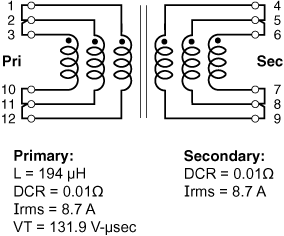## 站点设定

##### 货币设定

Cx Family Common Mode Chokes
0402CT Low Profile Chip Inductors
XAL7050 High-inductance Shielded Power Inductors
XGL4020 Ultra-low DCR Power Inductors
Quality

# Application example

for Hexa-Path – Forward

<- Back

## Formulas used to calculate electrical characteristics

Connecting windings in series:

• Inductance = Inductance(table) × (number of windings)2
• DCR = DCR(table) × number of windings
• Isat = (Isat(table) × 6) ÷ number of windings connected in series
• Irms = Irms(table)

Connecting windings in parallel:

• Inductance = Inductance(table)
• DCR = 1 ÷ [number of windings × (1 ÷ DCR(table))]
• Isat = (Isat(table) × 6) ÷ number of windings connected in series
• Irms = Irms(table) × number of windings

### Create a 130 Watt, 1:1, two switch forward converter transformer

Choose HPH6-2400L
Vin = 36 – 57 Vdc; Vout = 12 V, 10.8 A

Part number Inductance (µH) Percent Tolerance DCR max (Ω) Volt-time product (V-µs) Irms (A)
HPH6-2400L_ 194 25 0.03 131.9 2.9

Connecting primary windings in parallel:
When primary windings (Wpri) are connected in parallel, DCR
decreases, Irms increases, and inductance and volt-time product remain the same.

Example: For HPH6-2400L, connect three primary windings in parallel:
• Inductance = Inductance(table) = 194 μH
• DCR = 1 ÷ [Wpri × (1 ÷ DCR(table))] = 1 ÷ [(3 × (1 ÷ 0.030]) = 0.010 Ohms
• VT = VT(table) = 131.9 V-μsec
• Irms = Irms(table) × Wpri = 2.90 × 3 = 8.70 AConnecting secondary windings in parallel:
When secondary windings (Wsec) are connected in parallel, DCR decreases and Irms increases.

Example: For HPH6-2400L, connect three secondary windings in parallel:
• DCR = 1 ÷ [Wsec × (1 ÷ DCR(table))] = 1 ÷ [(3 × (1 ÷ 0.030)] = 0.010 Ohms
• Irms = Irms(table) × Wsec = 2.90 × 3 = 8.70 A